この節の作者: Rebecca Vederhus, Sebastian Jentschke

# From SPSS to jamovi: Analysis of frequencies¶

This comparison shows how a loglinear analysis is conducted in SPSS and jamovi. The SPSS test follows the description in chapter 19.9.2 in Field (2017), especially figure 19.7 and output 19.7 - 19.10. It uses the data set Cats and Dogs.sav which can be downloaded from the web page accompanying the book.
SPSS jamovi
In SPSS, you can run a loglinear analysis using: `Analyze``Loglinear``Model Selection`. In jamovi, this can be done using: `Analyses``Frequencies````Log- Linear Regression```.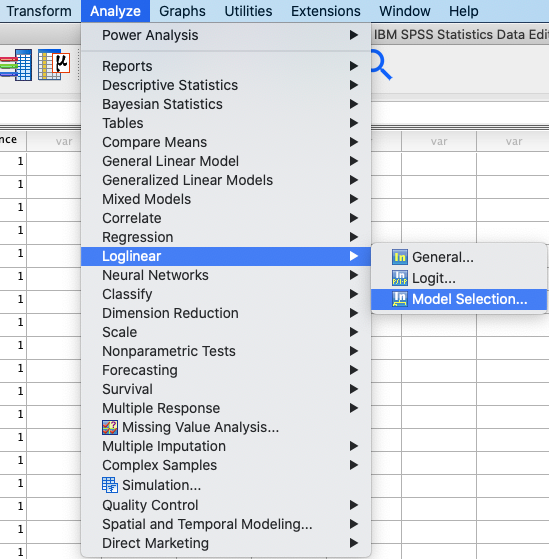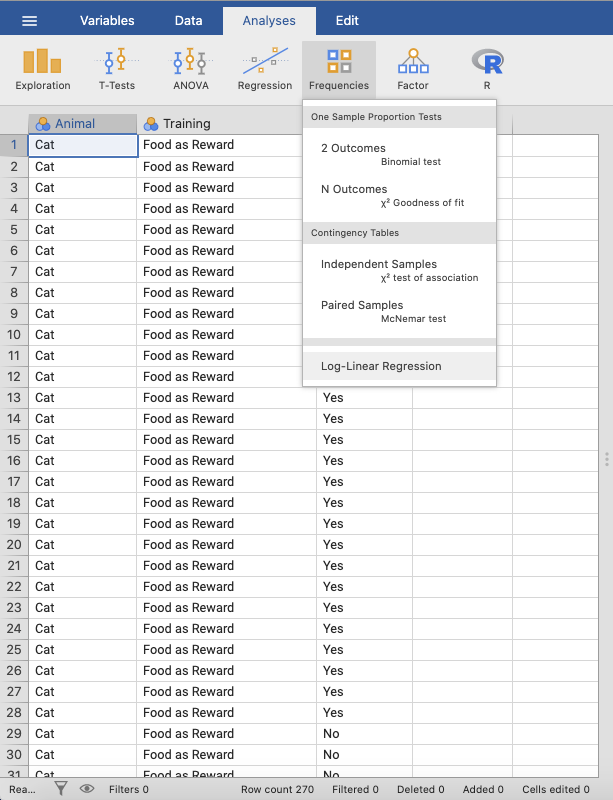In SPSS, move the variables `Animal`, `Training` and `Dance` to the `Factor(s)` box. Then, mark all three variables and click `Define Range`. In this window, set `Minimum` as 0 and `Maximum` as 1. Click `Continue`. In the box called `Model Building`, click ```Enter in single step```. In jamovi, move `Animal`, `Training` and `Dance` to `Factors`. Open the `Model Builder` window, click `+ Add New Block` and move the three variables to this block.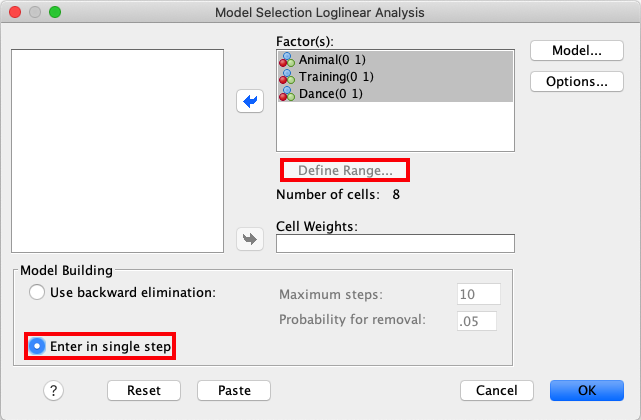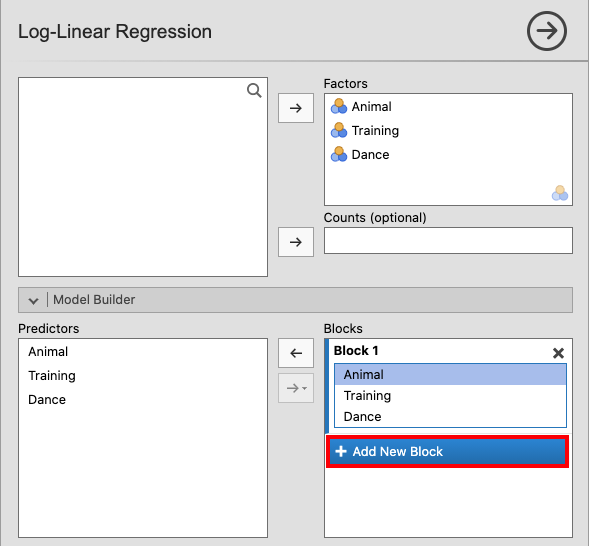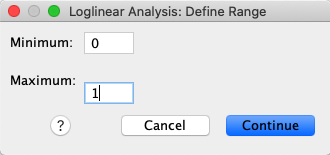Add another block. Mark all three variables and choose `All 2 way` from the drop-down menu. Then, add a third block and mark all three variables. Open the drop-down menu and click `All 3 way`.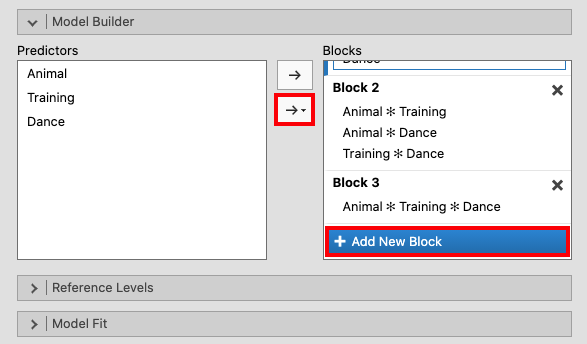Open `Model Fit` and tick the box for `Overall model test`. Lastly, tick `Likelihood ratio tests` in the `Model Coefficients` window.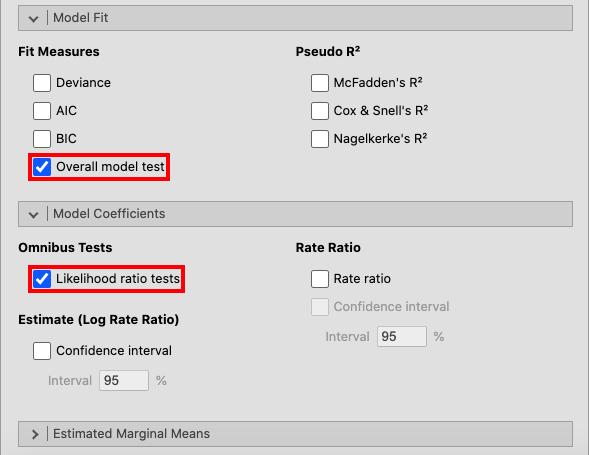Only the results in the output tables `K-Way and Higher-Order Effects` and `Partial Associations` in SPSS are replicated in the jamovi analysis.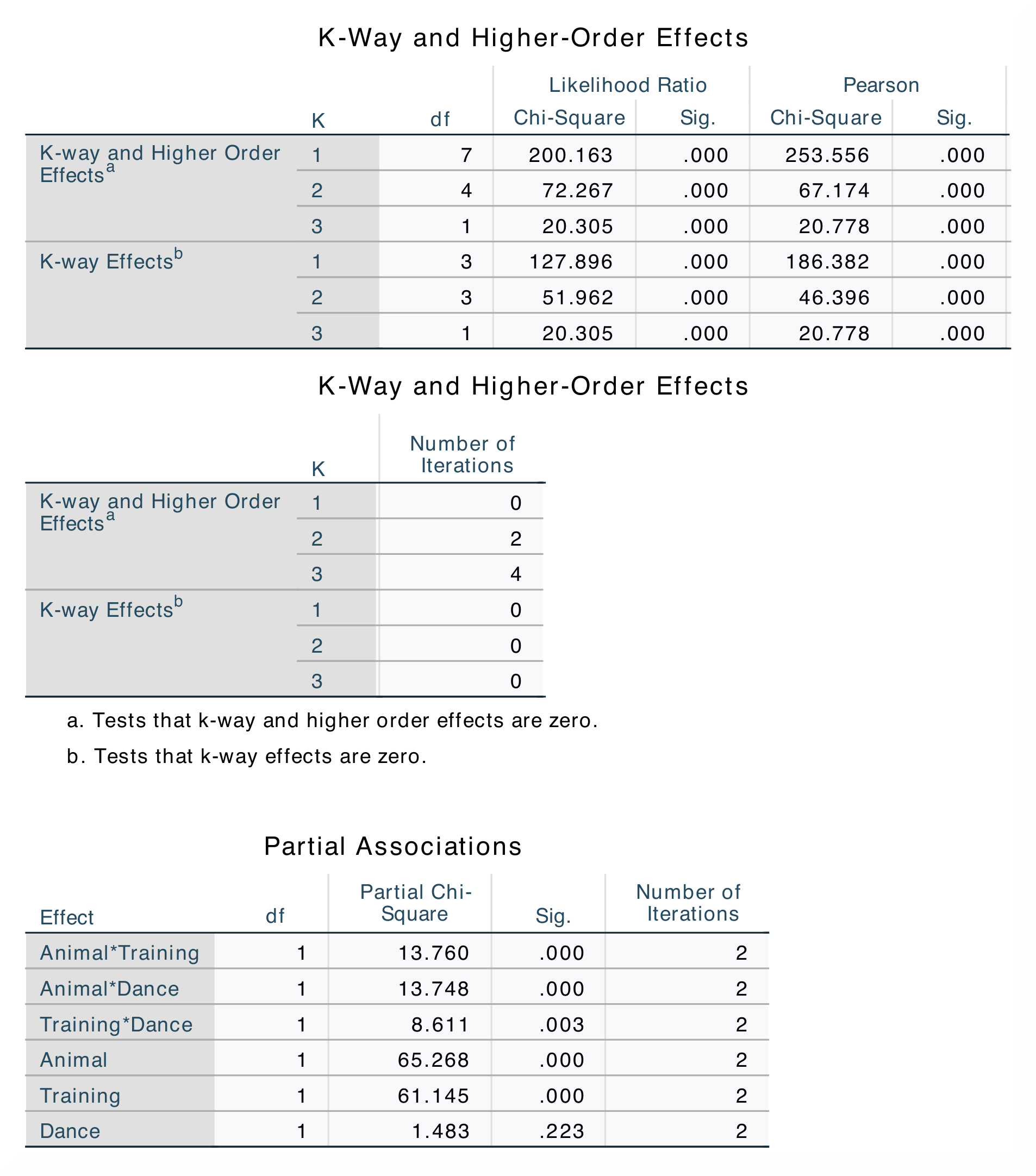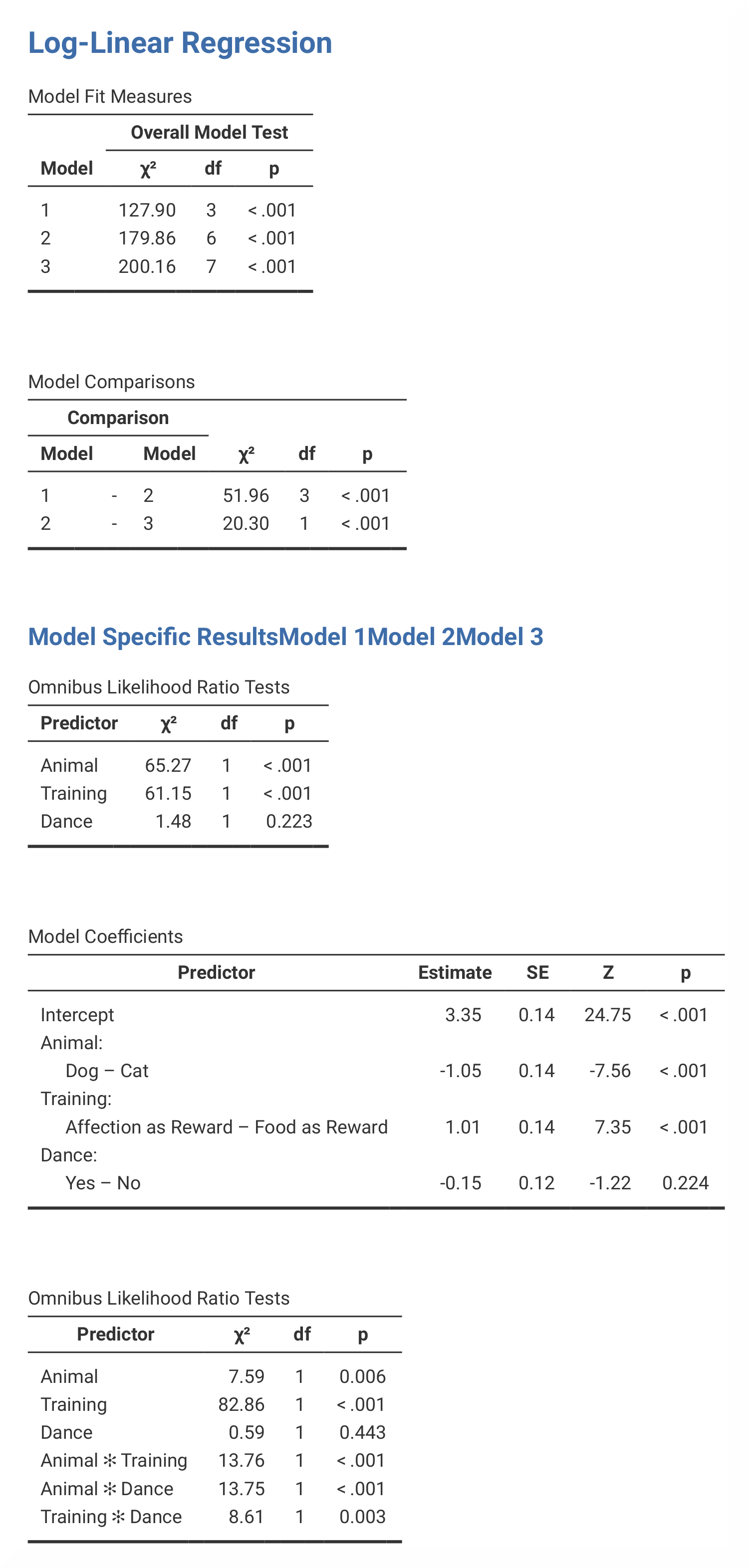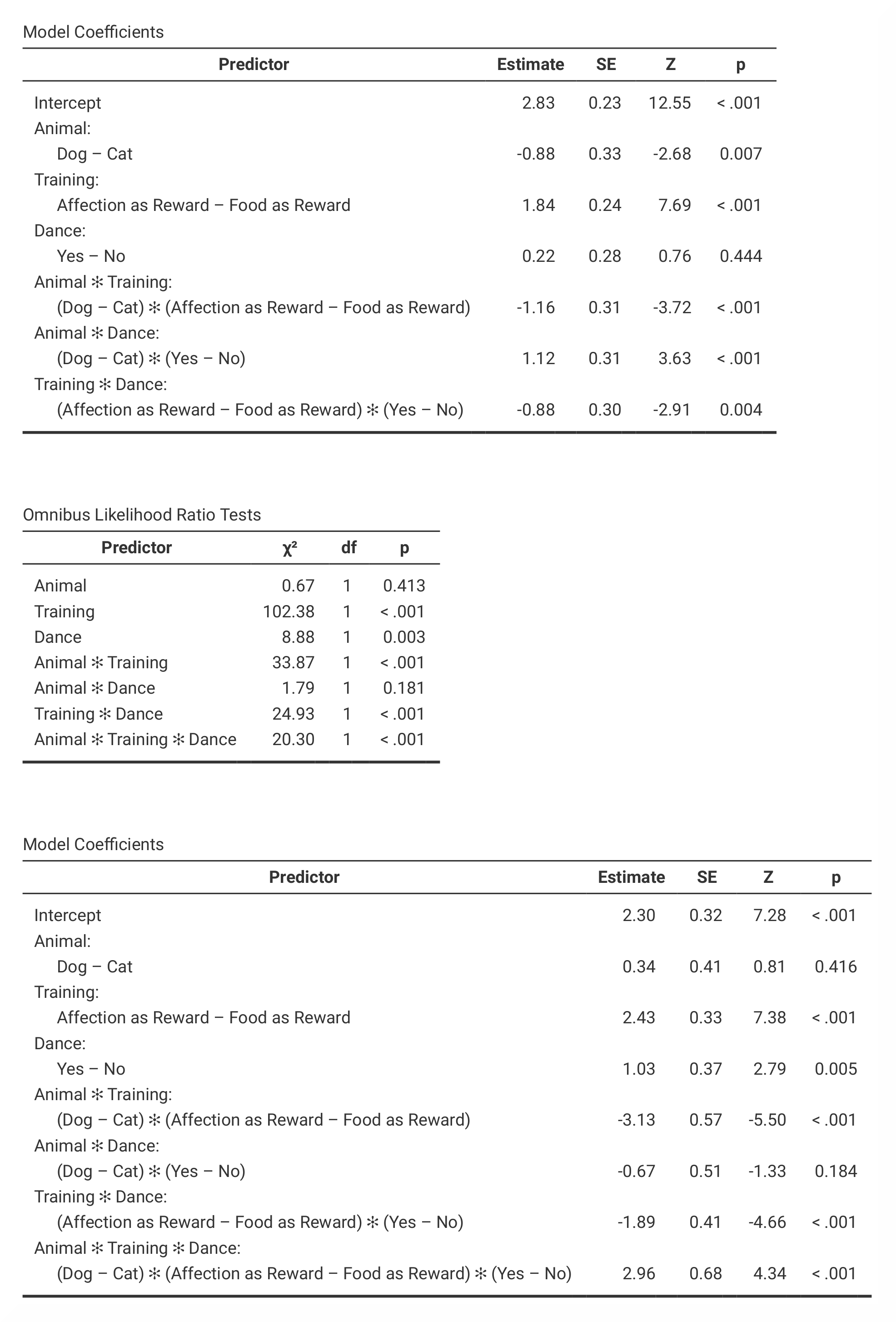In the `K-Way and Higher-Order Effects` table, you can find df-values, likelihood ratio statistics and significance values when K = 1, 2 and 3. The SPSS results also contains Pearson chi-square statistics. The different rows show if any higher-order effects or one-way effects significantly affect the model fit. The `Partial Associations` table breaks the model into specific parts and tells us which two-way interactions that significantly affect the fit of the model. You can tell this by looking at the significance values for the different interactions and comparing them. In jamovi, the values that are found in the ```K-Way and Higher-Order Effects``` table in SPSS can be found in the `Model Fit Measures` and `Model Comparisons` tables. However, jamovi does not provide Pearson chi-square statistics and the number of iterations. The partial associations table in jamovi is called `Omnibus Likelihood Ratio Tests` and are presented in three separate tables (one for each model).

Output from the SPSS analysis contains a lot of tables that are not included in the jamovi analysis. In addition, the results from the parameter estimates tables differ from each other, and are therefore not included here.

The numerical values for the statistics are the same: χ² = 127.90, p < .001; χ² = 200.16, p < .001; χ² = 51.96, p < .001: χ² = 20.30, p < .001; χ² = 65.27, p < .001; χ² = 61.15, p < .001; χ² = 1.48; χ² = 13.76, p < .001; χ² = 13.75, p < .001; χ² = 8.61, p < .01.

If you wish to replicate those analyses using syntax, you can use the commands below (in jamovi, just copy to code below to Rj). Alternatively, you can download the SPSS output files and the jamovi files with the analyses from below the syntax.
```HILOGLINEAR Animal(0 1) Training(0 1) Dance(0 1)
/CRITERIA ITERATION(20) DELTA(.0)
/PRINT=FREQ RESID ASSOCIATION ESTIM
/DESIGN.
```
```jmv::logLinear(
data = data,
factors = vars(Animal, Training, Dance),
blocks = list(
list("Animal", "Training", "Dance"),
list(c("Animal", "Training"), c("Animal", "Dance"),
c("Training", "Dance")),
list(c("Animal", "Training", "Dance"))),
refLevels = list(
list(var = "Animal", ref = "Cat"),
list(var = "Training", ref = "Food as Reward"),
list(var = "Dance", ref = "No")),
modelTest = TRUE,
dev = FALSE,
aic = FALSE,
pseudoR2 = NULL,
omni = TRUE)
```
SPSS output file containing the analyses jamovi file containing the analyses
References
Field, A. (2017). Discovering statistics using IBM SPSS statistics (5th ed.). SAGE Publications. https://edge.sagepub.com/field5e